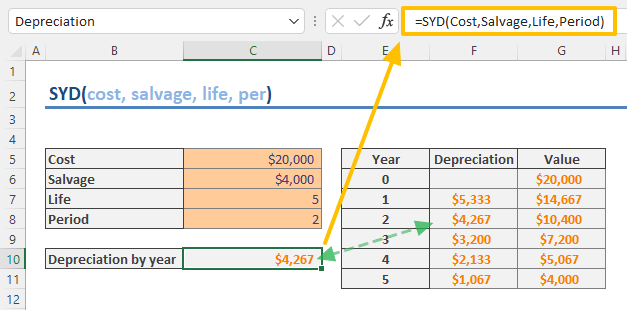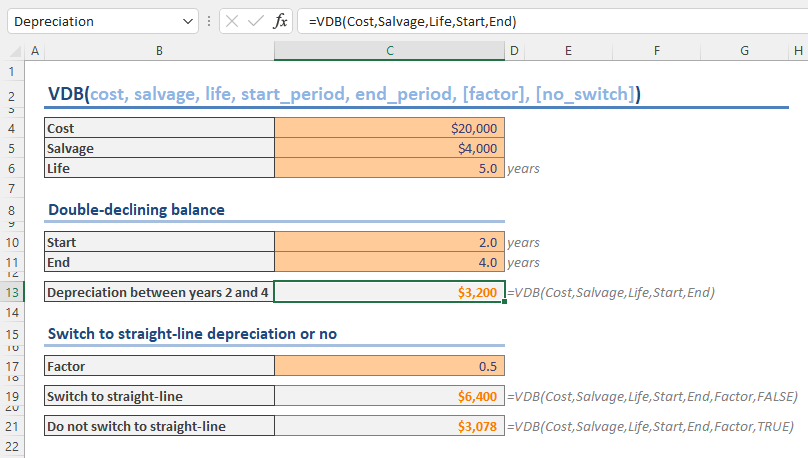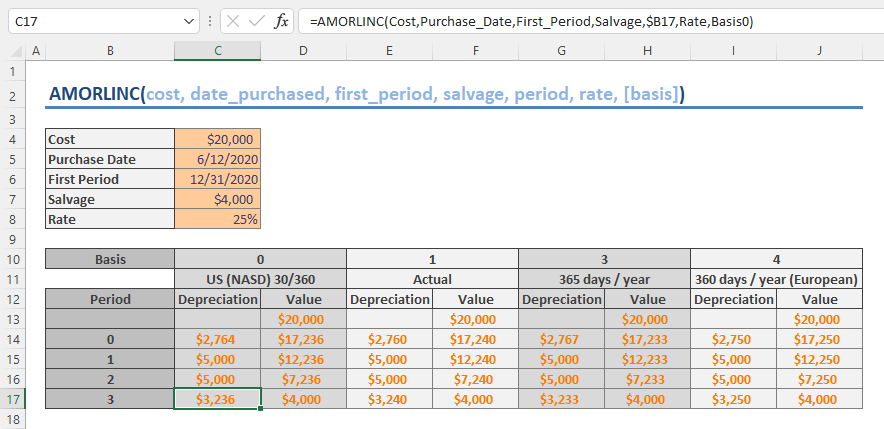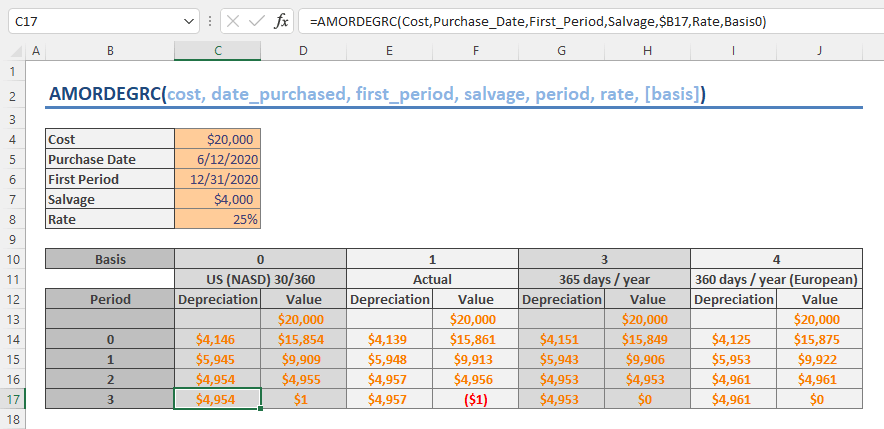Excel supports various methods and formulas to calculate depreciation. There 7 built-in functions dedicated to depreciation calculation. In this guide, we’re going to show you How to calculate depreciation in Excel in 7 ways.

## Straight line (SLN)

The straight line method assumes that asset's cost is depreciated the same amount for each accounting period. It is a popular method of calculating depreciation and amortization due to its simplicity.

Excel provides the SLN function for this method to calculate depreciation for a period. The function needs the initial and salvage costs of the asset as well as its useful life.

SLN(cost, salvage, life)

For an asset with an initial cost of \$20,000, a useful life of 5 years, and a salvage value of \$4,000, the formula should be as the following:

=SLN(20000,4000,5)## Sum-of-years' digits (SYD)

The sum-of-years' digits method is preferable if most of the depreciation associated with an asset is recognized in the first few years of its useful life. This approach is called accelerated depreciation as well.

You can use SYD function for this method to calculate the depreciation for a specified period. The function needs the initial and salvage costs of the asset and its useful life along with the period you want to see the numbers.

SYD(cost, salvage, life, per)

For an asset with an initial cost of \$20,000, a useful life of 5 years, and a salvage value of \$4,000, the formula for the 2nd year should be as the following:

=SYD(20000,4000,5,2)## Declining balance (DB)

The declining balance method is another way of calculating depreciation for the assets depreciated at a higher rate during initial years than in subsequent years. It is a useful approach for recording the depreciation of computers, cell phones, and other high-technology products that rapidly become obsolete.

Excel has the DB function to calculate the depreciation of an asset on the fixed-declining balance basis for a specified period. The function needs the initial and salvage costs of the asset, its useful life, and the period data by default. Additionally, you have an option to supply a month number in case the first year is partial.

DB(cost, salvage, life, per, [month])

For example, to calculate the depreciation for the 2nd year of an asset with an initial cost of \$20,000, a useful life of 5 years, and a salvage value of \$4,000, you can use the following:

Full year: =DB(20000,4000,5,2)

Partial year Starts at 7th month: =DB(20000,4000,5,2,7)## Double-declining balance (DDB)

The double declining balance method is one of the accelerated methods used when an asset's value depreciated at twice the rate of the straight line method.

The double declining balance method's function in Excel is the DDB. Different than the previous function, you can alter in what basis the DDB function calculates the depreciation. It can calculate the depreciation of an asset on the double-declining balance or another basis for a specified period.

The function needs the initial and salvage costs of the asset, its useful life, and the period data by default. Optionally, you can supply a factor value to determine the rate. If the factor should be omitted or entered as 2 to use double-declining method.

DDB(cost, salvage, life, period, [factor])

The following formula calculates the double-declining balance depreciation for the 3rd year of an asset with an initial cost of \$20,000, a useful life of 5 years, and a salvage value of \$4,000.

=DDB(20000,4000,5,3)## Variable-declining balance (VDB)

The variable-declining balance method is a combined method of the declining balance and the straight line approaches. The function starts depreciation calculation on declining balance basis and can switch to straight line depreciation when depreciation is greater than the declining balance calculation.

Excel's answer is the VDB function. Aside from the initial cost, salvage value and the useful life information, the VDB requires the dates of the start and end periods you want the depreciation for.

You can also populate the factor and no_switch arguments to alter the calculation method or eliminate the switching behavior.

VDB(cost, salvage, life, start_period, end_period, [factor], [no_switch])

For example, to calculate the depreciation between the 2nd and 4th years of an asset with an initial cost of \$20,000, a useful life of 5 years, and a salvage value of \$4,000, the formula should be:

=VDB(20000,4000,5,2,4)## French straight line (AMORLINC)

The French straight line or international straight line method is a form of a straight line depreciation, using custom rates and taking consider the exact days in a year.

Excel provides the AMORLINC function to calculate the depreciation for each accounting period, on a prorated basis. The prorated depreciation is considered when an asset is purchased in the middle of the accounting period.

The AMORLINC function requires initial cost of the asset as well as salvage value, the dates for the purchase and the end of the first period, the specific period to calculate the depreciation and the rate of the depreciation.

You can supply the basis argument to change the year basis.

AMORLINC(cost, date_purchased, first_period, salvage, period, rate, [basis])

In our example, an asset was purchased on June 12, 2020 at an initial cost of \$20,000. The end of the first period is December 31, 2020 and the depreciation rate is 20% per year with an expected salvage value of \$4,000.

=AMORLINC(20000,DATE(2020,12,6),DATE(2020,12,31),4000,2,0.25)## French declining balance (AMORDEGRC)

This is the declining balance, accelerated depreciation, method for the French accounting system. Excel provides the AMORDEGRC function for this type of depreciation calculation. The syntax is the same as in the straight line version, the AMORLINC.

AMORDEGRC(cost, date_purchased, first_period, salvage, period, rate, [basis])